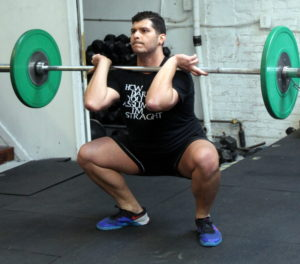## Friday, March 8, 2019

### Friday, March 8, 2019as many rounds as possible in 20 minutes:
400m run
20x dumbbell front rack walking lunge @ 45/30-lb. dbs
10x weighted ring dip @ 45/30-lb. db

### 1 Comment

1.–Scores–
Al C.: 3 rounds + 20x lunge (25-lbs. ring dip)
Hari P.: 4 rounds + 400m run (sub: bw rd)
Borja G.: 5 rounds + 3x lunge (sub: db. deficit pushup)
Charles B.: 5 rounds + 100m run (40-lb. dbs.; sub: bw rd)
Peter Mc.: 4 rounds + 200m run (35-lb. dbs.; sub: bw rd)
Neil A.: 4 rounds (25-lb. dbs. sub: row, db. rdl, 1/2x bw rd)
Anna G.: 4 rounds (20-lb. dbs.; sub: band rd)
Adrian P.: 3 rounds + 20x (35-lb. dbs.; sub: 7/10x bw rd)
Tommy W.: 4 rounds + 150m run (30-lb. dbs.; sub: 7/10x bw rd)
Zach D.: 4 rounds + 275m run (sub: bw rd)
Jerry I.: 4 rounds + 100m run (30-lb. dbs.; sub: band rd)
Dave R.: 4 rounds + 100m (sub: 7/10x rd)
Tarek K.: 3 rounds 375m (25-lb. dbs; sub: 7/10x band rd)
Amy S.: 4 rounds + 250m
Holmes H.: 4 rounds (20-lb. dbs; sub: band rd)
Tom G.: 3 rounds + 175m (15-lb. dbs; sub: pushup)
Chenelyn B.: 2 rounds + 200m (sub: bw lunge, incline pushup)
Andrew B.: 4 rounds + 10x lunge (25-lb. dbs; sub 7/10x band rd)
John N.: 4 rounds + 100m (30-lb. dbs; sub: 1/2x bw rd)
Alissa G.: 4 rounds + 100m (sub: 1/2x bw rd)
Diana G.: 4 rounds + 15x lunge (20-lb. dbs; sub: russian swing w 35-lb. kb)
Hirofumi J.: 4 rounds (20-lb. dbs; sub: band rd)
JP S.: 4 rounds (35-lb. dbs; sub: 20-cal bike, 1/2x band rd)
Michael Fo.: 4 rounds + 1x lunge (30-lb. dbs; sub: 7/10x band rd)
Mead O.: 3 rounds + 200m (10-lb. dbs; sub: 200m run, 1/2x incline pushup)
Kate G.: 4 rounds (25-lb. dbs; sub: 7/10x band rd)
Alli P.: 4 rounds + 10x lunge (25-lb. dbs; sub: 7/10x bw rd)
Kevin M.: 4 rounds (20-lb. dbs; sub: pushup)
Joya TD.: 4 rounds + 100m (25-lb. dbs; sub: 1/2x bw rd)
Josh M.: 3 rounds + 10x rd (40-lb. dbs, 15-lb. rd)
Andrew Z.: 4 rounds + 75m (15-lb. rd)
Chris H.: 3 rounds + 6x dip (30-lb. rd)
Mel F.: 3 rounds + 400m (sub: bw rd)
Katie G.: 4 rounds + 100m (20-lb. dbs; sub: 7/10x band rd)
Cristian D.: 4 rounds (25-lb. dbs; sub: 7/10x band rd)
Charlie S.: 4 rounds (35-lb. dbs, 15-lb. rd)
Aaron B.: 4 rounds + 300m (35-lb. dbs; sub: 7/10x band rd)
Emmy H.: 4 rounds + 7x pushup (sub: pushup)
Simon B.: 4 rounds + 60m (20-lb. dbs; sub: 7/10x band rd)
Francisco L.: 4 rounds (40-lb. dbs; sub: bw rd)
Adrienne V. 4 rounds (15-lb. dbs; sub: band rd)
Bill G.: 4 rounds (sub: 25-lb. rd)
Collin S.: 4 rounds + 330m (30-lb. dbs; sub: bw rd)
Mark Co.: 3 rounds + 150m (35-lb. dbs; sub: 15-lb rd)
Seth B.: 3 rounds + 400m (35-lb. dbs; sub: 2x push-up)
Seth C.: 3 rounds + 300m (30-lb. dbs; sub: farmers, bw rd)
Lily S.: 4 rounds (20-lb. dbs; sub: knee push-up)
Krystin H.: 4 rounds + 78m (10-lb. dbs; sub: push-up/knee push-up)
Jason S.: 4 rounds + 150m (30-lb. dbs; sub: 1/2x bw rd)
Jason Y.: 4 rounds (25-lb. dbs; sub: bw rd/push-up)
Matt K.: 3 rounds + 5 lunges (35-lb. dbs; sub: band rd)
Julian M.: 4 rounds + 250m (20-lb. dbs; sub: band rd)
Michael H.: 6 rounds (walking lunge; sub: bw rd, 90″ bike)
Charlie K.: 3 rounds + 15 lunges (40-lb. dbs; sub bw rd)